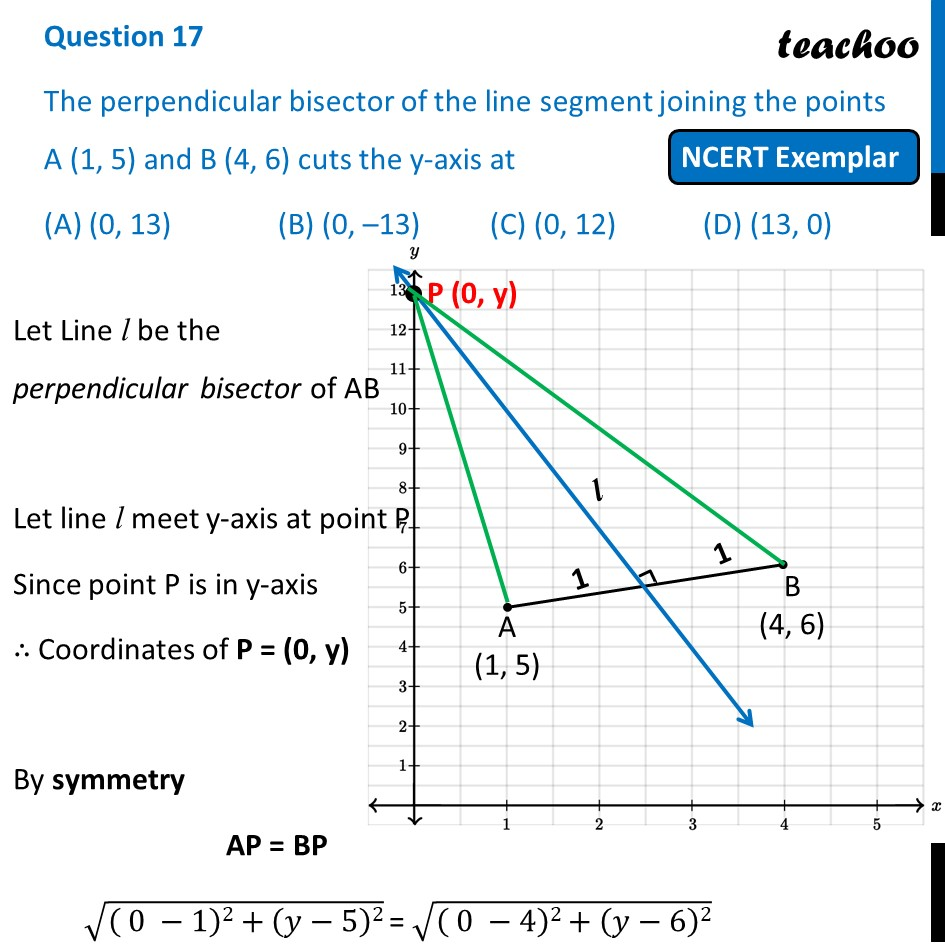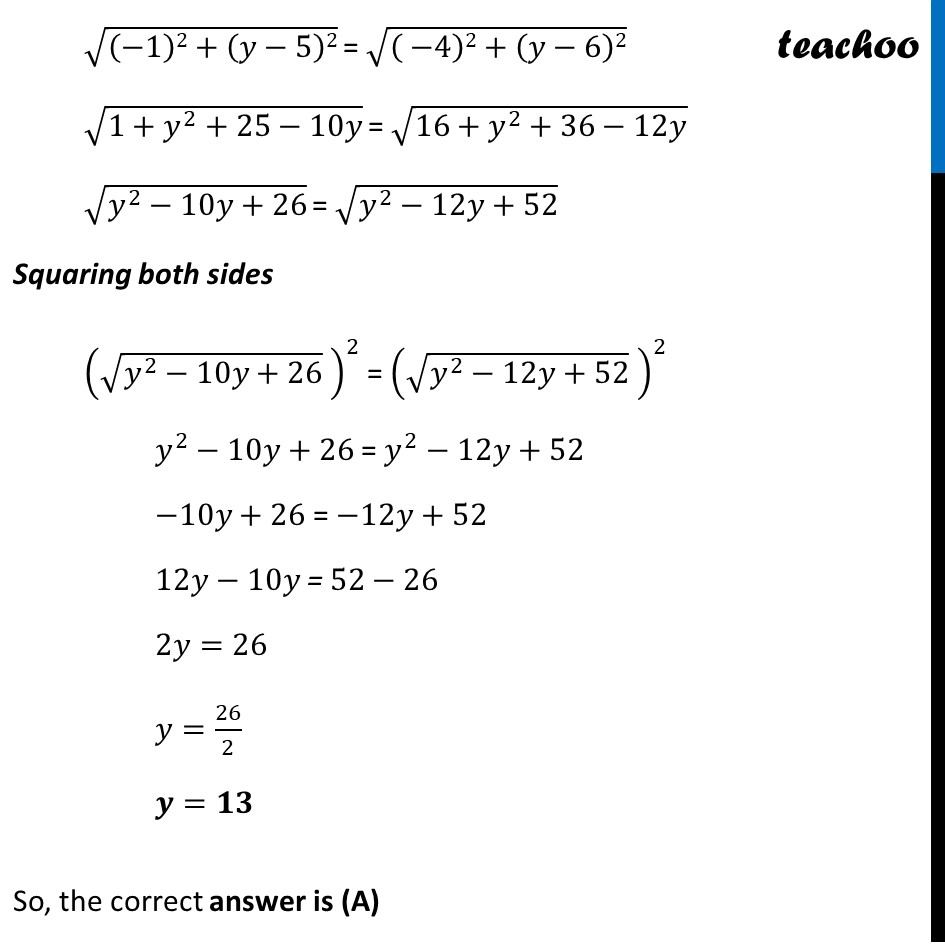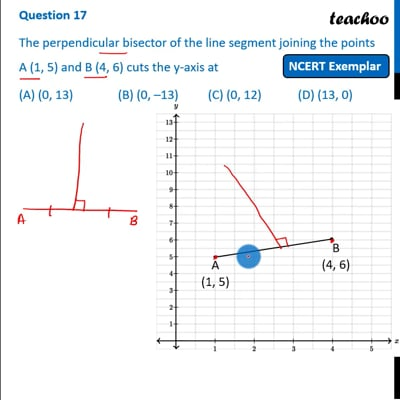NCERT Exemplar - MCQ

Chapter 7 Class 10 Coordinate Geometry
Serial order wise

## (A) (0, 13)      (B) (0, –13)     (C) (0, 12)      (D) (13, 0)This video is only available for Teachoo black users

Maths Crash Course - Live lectures + all videos + Real time Doubt solving!

### Transcript

Question 17 The perpendicular bisector of the line segment joining the points A (1, 5) and B (4, 6) cuts the y-axis at (A) (0, 13) (B) (0, –13) (C) (0, 12) (D) (13, 0) Let Line l be the perpendicular bisector of AB Let line l meet y-axis at point P Since point P is in y-axis ∴ Coordinates of P = (0, y) By symmetry AP = BP √(( 0 −1)2+(𝑦−5)2) = √(( 0 −4)2+(𝑦−6)2) √((−1)2+(𝑦−5)2) = √(( −4)2+(𝑦−6)2) √(1+𝑦^2+25−10𝑦) = √(16+𝑦^2+36−12𝑦) √(𝑦^2−10𝑦+26) = √(𝑦^2−12𝑦+52) Squaring both sides (√(𝑦^2−10𝑦+26) )^2 = (√(𝑦^2−12𝑦+52) )^2 𝑦^2−10𝑦+26 = 𝑦^2−12𝑦+52 −10𝑦+26 = −12𝑦+52 12𝑦−10𝑦 = 52−26 2𝑦=26 𝑦=26/2 𝒚=𝟏𝟑 So, the correct answer is (A)